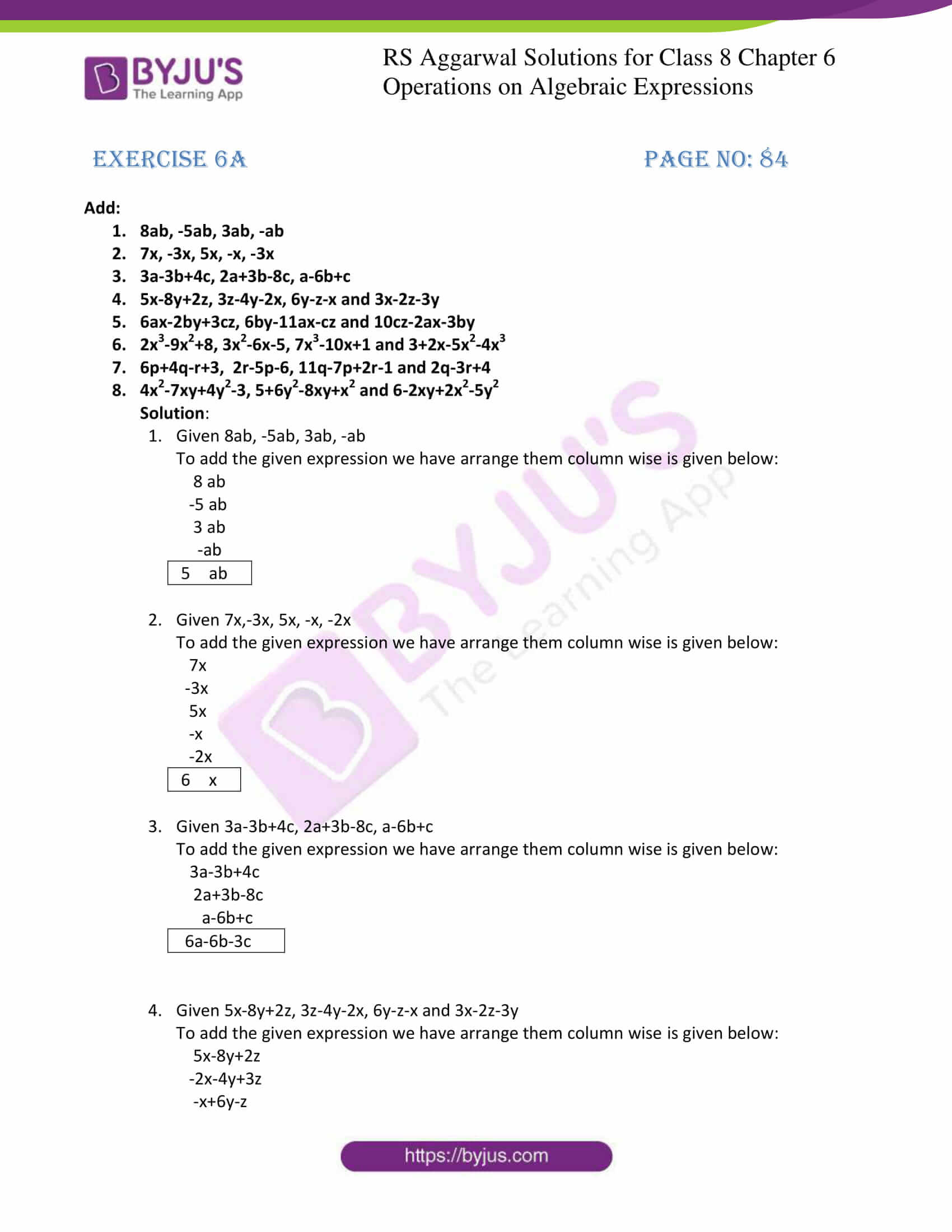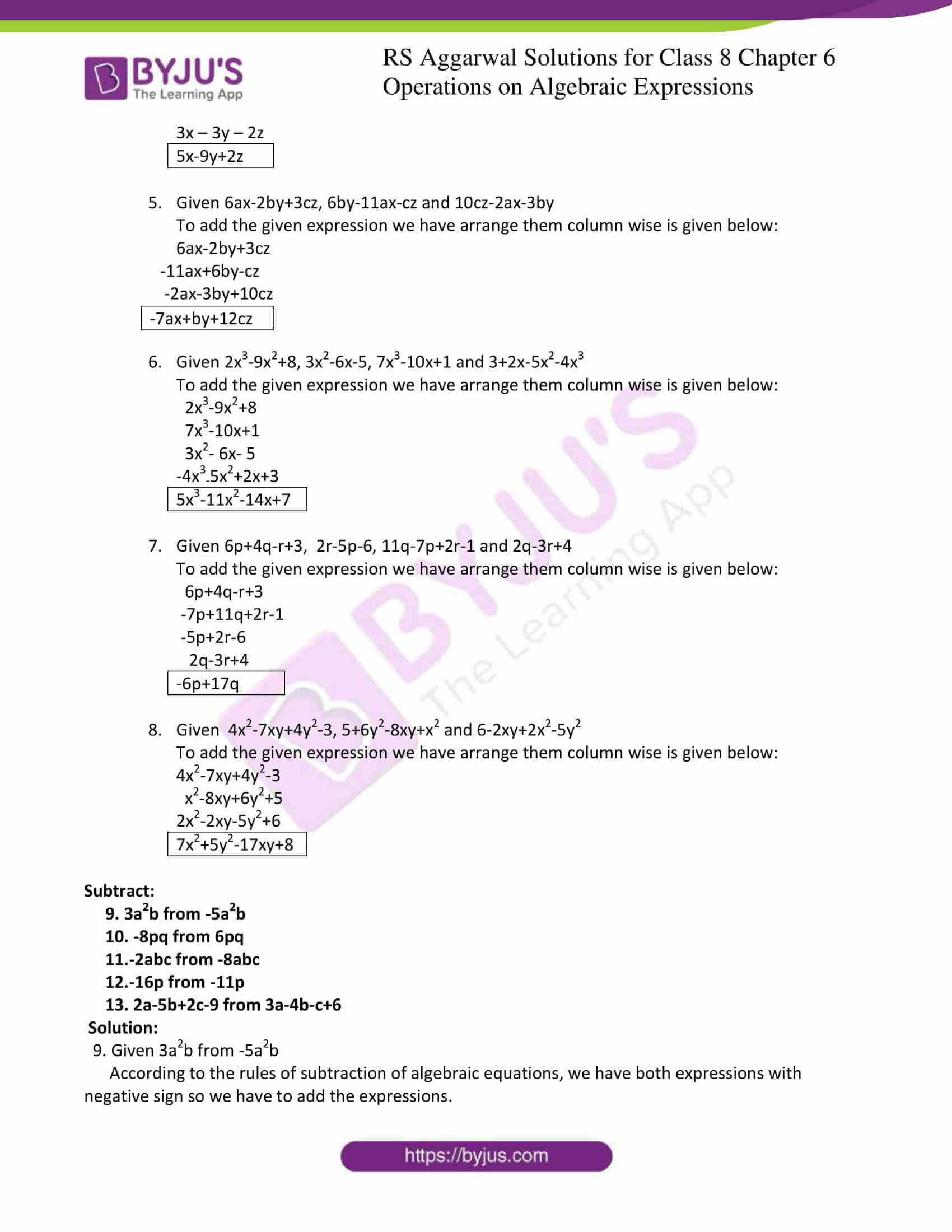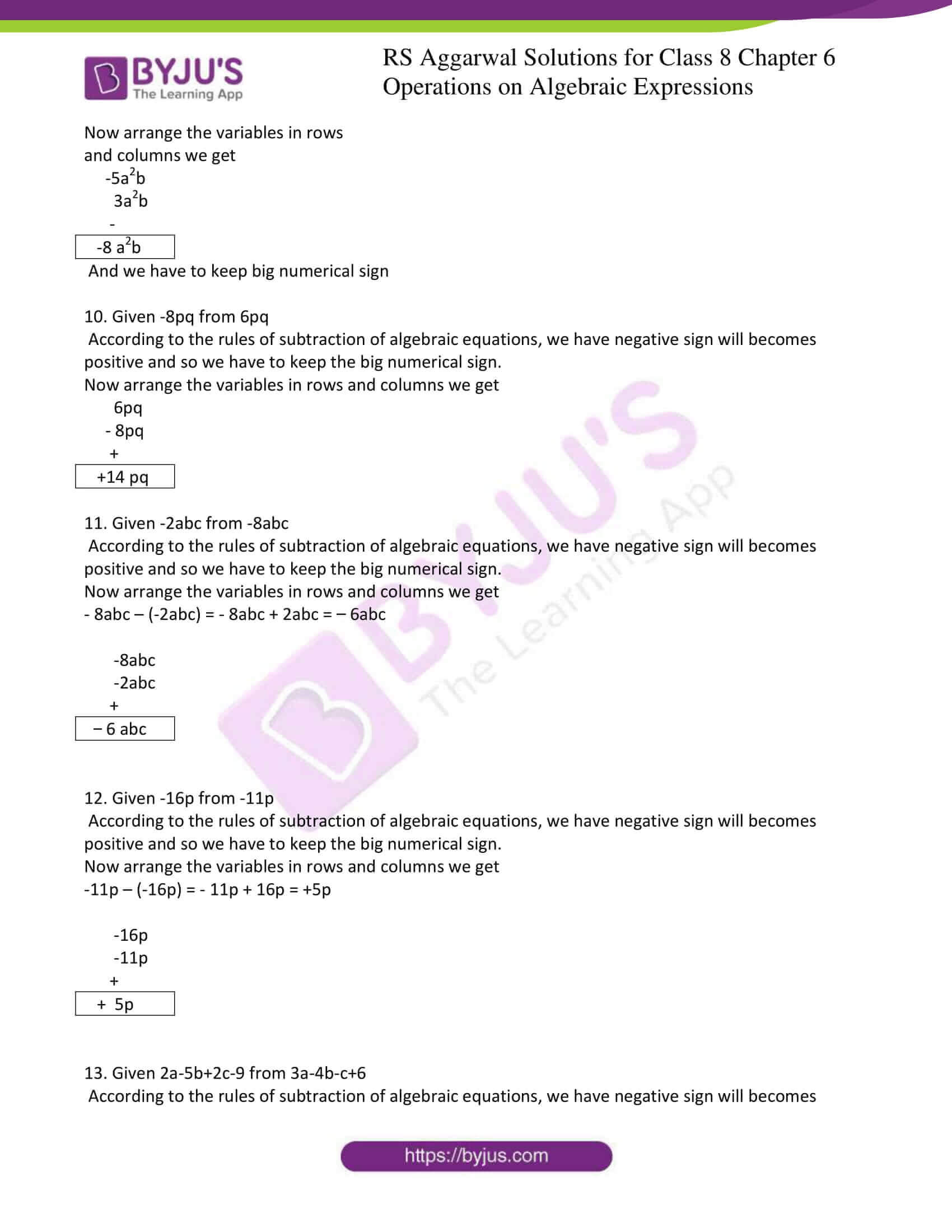# RS Aggarwal Solutions for Class 8 Maths Chapter 6 - Operations on Algebraic Expressions Exercise 6A

Students can refer and download the RS Aggarwal Solutions for the Exercise 6A of Class 8 Maths Chapter 6, Operations on Algebraic Expressions from the link available here. The questions present in this have been solved by BYJU’S experts in Maths, and this will help students solve the problems without any difficulties. In this exercise, we shall study the addition and subtraction of algebraic expressions. By practising sincerely the students can obtain worthy results in the Class 8 exams by using the RS Aggarwal Solutions.

By practising the RS Aggarwal Solutions for class 8, students will be able to grasp the concepts perfectly. Hence, students whose aim is to score high in the examinations are advised to go through RS Aggarwal Solutions for Class 8.

## Download PDF of RS Aggarwal Solutions for Class 8 Maths Chapter 6 – Operations on Algebraic Expressions Exercise 6A### Access answers to Maths RS Aggarwal Solutions for Class 8 Chapter 6 – Operations on Algebraic Expressions Exercise 6A

1. 8ab, -5ab, 3ab, -ab

2. 7x, -3x, 5x, -x, -3x

3. 3a-3b+4c, 2a+3b-8c, a-6b+c

4. 5x-8y+2z, 3z-4y-2x, 6y-z-x and 3x-2z-3y

5. 6ax-2by+3cz, 6by-11ax-cz and 10cz-2ax-3by

6. 2x3-9x2+8, 3x2-6x-5, 7x3-10x+1 and 3+2x-5x2-4x3

7. 6p+4q-r+3, 2r-5p-6, 11q-7p+2r-1 and 2q-3r+4

8. 4x2-7xy+4y2-3, 5+6y2-8xy+x2 and 6-2xy+2x2-5y2

Solution:

1. Given 8ab, -5ab, 3ab, -ab

To add the given expression we have arrange them column wise is given below:

8 ab

-5 ab

3 ab

-ab

 5ab

2. Given 7x,-3x, 5x, -x, -2x

To add the given expression we have arrange them column wise is given below:

7x

-3x

5x

-x

-2x

 6x

3. Given 3a-3b+4c, 2a+3b-8c, a-6b+c

To add the given expression we have arrange them column wise is given below:

3a-3b+4c

2a+3b-8c

a-6b+c

 6a-6b-3c

4. Given 5x-8y+2z, 3z-4y-2x, 6y-z-x and 3x-2z-3y

To add the given expression we have arrange them column wise is given below:

5x-8y+2z

-2x-4y+3z

-x+6y-z

3x – 3y – 2z

 5x-9y+2z

5. Given 6ax-2by+3cz, 6by-11ax-cz and 10cz-2ax-3by

To add the given expression we have arrange them column wise is given below:

6ax-2by+3cz

-11ax+6by-cz

-2ax-3by+10cz

 -7ax+by+12cz

6. Given 2x3-9x2+8, 3x2-6x-5, 7x3-10x+1 and 3+2x-5x2-4x3

To add the given expression we have arrange them column wise is given below:

2x3-9x2+8

7x3-10x+1

3x2– 6x- 5

-4x3-5x2+2x+3

 5x3-11x2-14x+7

7. Given 6p+4q-r+3, 2r-5p-6, 11q-7p+2r-1 and 2q-3r+4

To add the given expression we have arrange them column wise is given below:

6p+4q-r+3

-7p+11q+2r-1

-5p+2r-6

2q-3r+4

 -6p+17q

8. Given 4x2-7xy+4y2-3, 5+6y2-8xy+x2 and 6-2xy+2x2-5y2

To add the given expression we have arrange them column wise is given below:

4x2-7xy+4y2-3

x2-8xy+6y2+5

2x2-2xy-5y2+6

 7x2+5y2-17xy+8

Subtract:

9. 3ax2b from -5ax2b

10. -8pq from 6pq

11.-2abc from -8abc

12.-16p from -11p

13. 2a-5b+2c-9 from 3a-4b-c+6

Solution:

9. Given 3ax2b from -5ax2b

According to the rules of subtraction of algebraic equations, we have both expressions with negative sign so we have to add the expressions.

Now arrange the variables in rows and columns we get

-5ax2b

3ax2b

 -8 ax2b

And we have to keep big numerical sign

10. Given -8pq from 6pq

According to the rules of subtraction of algebraic equations, we have negative sign will becomes positive and so we have to keep the big numerical sign.

Now arrange the variables in rows and columns we get

6pq

– 8pq

+

 +14 pq

11. Given -2abc from -8abc

According to the rules of subtraction of algebraic equations, we have negative sign will becomes positive and so we have to keep the big numerical sign.

Now arrange the variables in rows and columns we get

– 8abc – (-2abc) = – 8abc + 2abc = – 6abc

-8abc

-2abc

+

 -6 abc

12. Given -16p from -11p

According to the rules of subtraction of algebraic equations, we have negative sign will becomes positive and so we have to keep the big numerical sign.

Now arrange the variables in rows and columns we get

– 11p – (-16p) = – 11p + 16p = 5p

-16p

-11p

+

 + 5p

13. Given 2a-5b+2c-9 from 3a-4b-c+6

According to the rules of subtraction of algebraic equations, we have negative sign will becomes positive and so we have to keep the big numerical sign.

Now arrange the variables in rows and columns we get

3a-4b-c+6

2a-5b+2c-9

– +- +

 + a+b-3c+15

Exercise 6B

Exercise 6C

Exercise 6D

Exercise 6E

## RS Aggarwal Solutions for Class 8 Maths Chapter 6 – Operations on Algebraic Expressions Exercise 6A

Exercise 6A of RS Aggarwal Solutions for Chapter 6, Operations on Algebraic Expressions deals with the basic concepts related to Algebraic Expressions. We can say that this exercise mainly deals with the operations on given Algebraic Expressions that the students have learned in this chapter. Some of the topics focused prior to exercise 6A include the following.